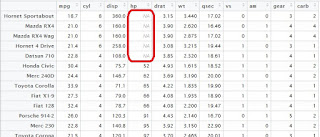## Pages

### R Tutorial 8.0

This is short blog that covers the sorting procedure in R.

I won't write much just to fill in the space, better you go inside, as learn sorting !

Let's use an inbuilt dataset in R for understanding sorting.

### Data_1   = mtcars Sorting a Vector

Since vector is uni-variate data i.e. contains only one column, so it doesn't require mentioning a sorting variable.

HP_sorted_asc = sort(Data_1\$hp)
HP_sorted_dsc = sort(Data_1\$hp, decreasing = TRUE)

Option decreasing is TRUE be default !

### Sorting a Data Frame

# First we attach the data, in order to use the column names of the data directly

attach(Data_1)

# Let's sort the data in the increasing order of weight (wt)
Data_sorted = Data_1[order(wt), ]

# Let's now sort the data in the increasing order of Horse power (hp) and weight (wt)
Data_sorted = Data_1[order(hp,wt), ]

# For changing the default ascending order to descending order, put a minus (-) sign before
# column name
Data_sorted = Data_1[order(hp,-wt), ]

### What if there are missing values in sorting column

First, we create missing values for demo :

rm(list = ls())                           # Let's clear work space
Data_1   = mtcars

# Suppose, we create few missing values in hp column
Data_1[1:5,4] = NA

# I am jumbling the missing values, for testing purpose

attach(Data_1)
Data_new = Data_1[order(mpg),]
detach(Data_1)
rm(Data_1)

# would now use the Data_new, which has missing values in hp column, that too jumbed
attach(Data_new)

# Let's now sort the data on the basis of column having missing values
Data_sorted_1 = Data_new[order(hp),]
Data_sorted_2 = Data_new[order(hp, na.last=TRUE),]
Data_sorted_3 = Data_new[order(hp, na.last=FALSE),]
Data_sorted_4 = Data_new[order(hp, na.last=NA),]

### Let's see how the results differ :

Data_sorted_1 :  Data is sorted on hp column in ascending order of hp and all the observations with missing values are left in the last. Even if we consider the descending order while sorting, the observations with missing values in the sorting column by default are left in the end of dataset.

Data_sorted_2 :  Data is same as Data_sorted_1, the only difference is in this statement we have instructed to keep the observation with missing values in the sorting column in the last.Data_sorted_3
Data_sorted_3 :  In this code, we have instructed R to keep the observation with missing values in the sorting column in the starting, and R obeys.Data_sorted_4 :  In this code, we have instructed R to drop all the observations with missing values in the sorting column, Hence all the 5 observations that have missing hp are deleted.

Enjoy reading our other articles and stay tuned with us.

Kindly do provide your feedback in the 'Comments' Section and share as much as possible.